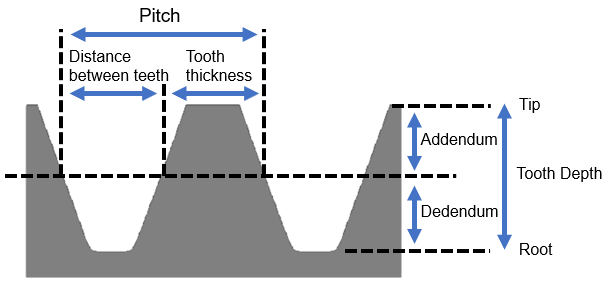## Gear CalculationsDedendum = 1.167 x Module
= 1.167 x

Tooth Depth = 2.167 x Module
= 2.167 x

Radius of Tooth Root = 0.3 x Module
= 0.3 x

Pitch = Distance between teeth + Tooth thickness
= +

Distance between teeth = 0.5 x Pitch
= 0.5 x

Tooth thickness = 0.5 x Pitch
= 0.5 x

Circular Pitch = Pi x Module
= Pi x

Diametral Pitch = 25.4 / Module
= 25.4 /

Reference Diameter = Module x Number of Teeth
= x

Reference Diameter = (Pitch / Pi) x Number of Teeth
= ( / Pi) x

Tip Diameter = Reference Diameter + 2 x Module
= + 2 x

Tip Diameter = Module x (Number of Teeth + 2)
= x (+2)

Root Diameter = Reference Diameter - 2.5 x Module
= - 2.5 x

If you have any suggestions please let us know at: suggestionsfeed@gmail.com